Related Articles
Contingency Table in Python
• Last Updated : 21 Jan, 2019

Estimations like mean, median, standard deviation, and variance are very much useful in case of the univariate data analysis. But in the case of bivariate analysis(comparing two variables) correlation comes into play.

Contingency Table is one of the techniques for exploring two or even more variables. It is basically a tally of counts between two or more categorical variables.

 `import` `numpy as np ` `import` `pandas as pd ` `import` `matplotlib as plt `

 `data ``=` `pd.read_csv(``"loan_status.csv"``) ` ` `  `print` `(data.head(``10``)) `

Output: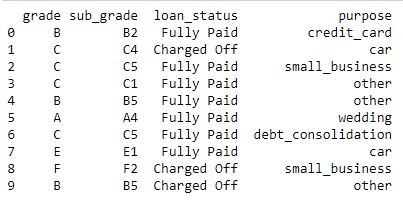Describe Data

 `data.describe() `

Output: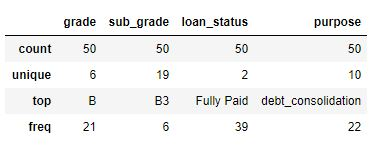Data Info

 `data.info() `

Output: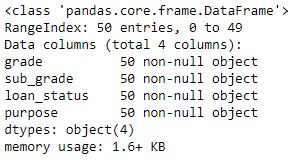Data Types

 `# data types of feature/attributes  ` `# in the data ` `data.dtypes `

Output: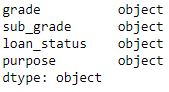Code #1: Contingency Table showing correlation between Grades and loan status.

 `data_crosstab ``=` `pd.crosstab(data[``'grade'``], ` `                            ``data[``'loan_status'``],  ` `                               ``margins ``=` `False``) ` `print``(data_crosstab) `

Output: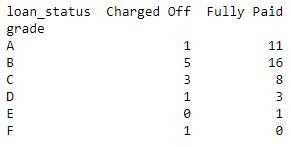Code #2: Contingency Table showing correlation between Purpose and loan status.

 `data_crosstab ``=` `pd.crosstab(data[``'purpose'``],  ` `                            ``data[``'loan_status'``], ` `                                ``margins ``=` `False``) ` `print``(data_crosstab) `

Output: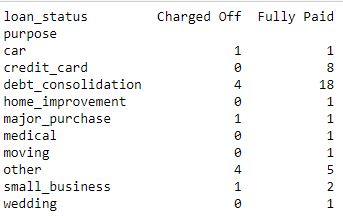Code #3: Contingency Table showing correlation between Grades+Purpose and loan status.

 `data_crosstab ``=` `pd.crosstab([data.grade, data.purpose],  ` `                             ``data.loan_status, margins ``=` `False``) ` `print``(data_crosstab) `

Output: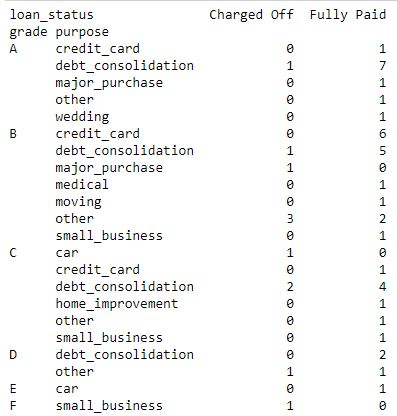So as in the code, Contingency Tables are giving clear correlation values between two and more variables. Thus making it much more useful to understand the data for further information extraction.
.My Personal Notes arrow_drop_up
Recommended Articles
Page :